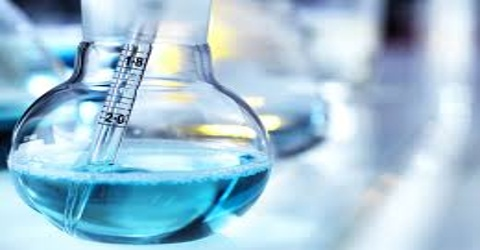# Units of Concentration

Units of Concentration

Whenever the concentration of a solution is reported, the appropriate unit in which it is given is also mentioned. In chemical literature the following units are used:

(i) Molarity ( mol L-1): The molarity of a solution is the number of moles of the solute dissolved in 1000 mL of the solution. Thus if 60 g (one mol) of urea is dissolved in water to form a solution occupying a volume of 1000 ml. at a specified temperature, the solution is said to be 1.0 molar. 6.0 g of urea per 1000 ml of solution will give a 0.1 molar solution whereas 0.6 g of urea per 1000 ml of solution will give a 0.01 molar solution. Since the volume of the solution will depend on the temperature, molarity will vary with temperature.

(ii) Molality (m): Molality of a solution is the number of moles of the solute dissolved in 1000 g of the solvent. In other words, if one mol of a substance is dissolved in 1000 g of the solvent, the solution is said to be 1.0 molal. 60 g of urea dissolved in 1000 g of water gives a 1.0 molal solution. Molality is independent of temperature.

(iii) Mole fraction: Mole fraction of the solute is defined as the ratio of the number of moles of the solute to the total number of moles of the solute and solvent taken together. If n be the number of moles of solute and N the number of moles of the solvent then the mole fraction of the solute is: n / (n+N).

Taking the previous example, if 60 g of urea is dissolved in 1000 g of water, then n = 60/60 and N = 1000/18 = 55.56. Therefore, mole friction of urea in the solution is 1/(1+55.56). The mole fraction of water can be easily calculated. Thus it is equal to, 55.56/(1+55.56) = 0.9823. It is to be noted that in any solution the sum of the mole fractions of solute and solvent is always equal to unity. Mole fraction is a dimensionless quantity and represents a pure number. When mole fraction is multiplied by 100 it is called mole percent. Mole fraction is independent of temperature. If w is the mass of a substance of molecular mass m and if it is dissolved in Wg of a solvent of molecular mass M then,

Mole fraction of the solute X2 = [(w/m) / (w/m) + (W/M)]

Mole fraction of the solvent X1 = [(W/M) / (w/m) + (W/M)]

(iv) Normality (N): It is another concentration unit used to be in use in the past. It was defined as. “The number of equivalent mass of a substance present in 1 L of a solution.” Normality is also temperature dependent. However, normality is no longer used in chemical calculations and can be found only in older text.